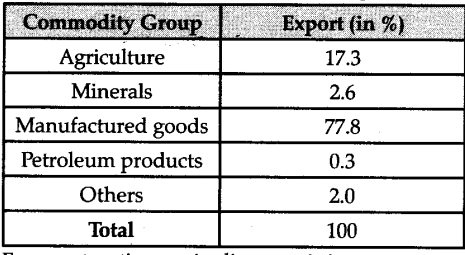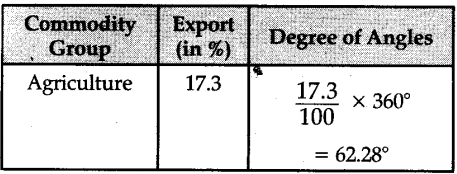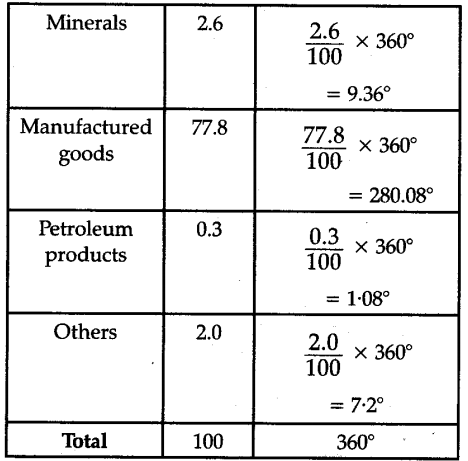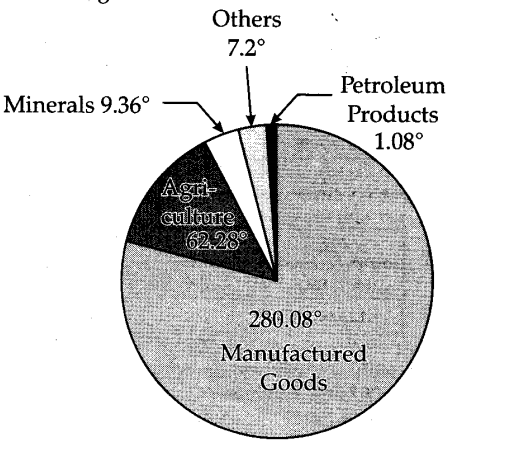# Percentage distribution of export of India is given below. Construct a pie diagram using this data:

Percentage distribution of export of India is given below. Construct a pie diagram using this data:For constructing a pie diagram, it is necessary to convert the percentage into angles of different degrees. This conversion is shown below :pie diagram showing percentage of export of data is given below :